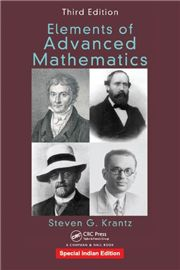# ELEMENTS OF ADVANCED MATHEMATICS, 3/e STEVEN G. KRANTZ-S.CHAND

595.00 535.00

• Basic Logic
• Methods of Proof
• Set Theory
• Relations and Functions
• Axioms of Set Theory, Paradoxes, and Rigor
• Number Systems
• More on the Real Number System
• A Glimpse of Topology
• Theoretical Computer Science
• The P/NP Problem
• Examples of Axiomatic Theories
• Zero-Knowledge Proofs
• Solutions to Selected Exercises